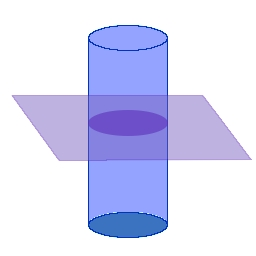# Visualisation of linear MHD waves in a coronal loopA loop modeled as a straight cylinder
##### By Erwin Verwichte

The theory of MHD waves in loops has been modeled by Edwin & Roberts (1983), where the loop is considered to be a straight cylinder with the equilibrium media inside and outside homogeneous. Across the interface, total pressure and radial displacement are continuous. The magnetic field is directed parallel to the cylinder axis.

To help understand how the linear MHD wave solutions look like, I have produced images and movies of the density perturbation and velocity, as well as dispersion diagrams for a typical coronal case (normalised w.r.t. internal sound speed):

CS,0 = 1.00, VA,0 = 2.00, CS,e = 0.50, VA,e = 5.00,e /0 = 0.2, Be / B0 = 1.00, Te / T0 = 0.250 = 0.30,e = 0.01,

## Spatial structure of the modesTransverse structureLongitudinal structure The following cuts are taken of the cylinder

### m=5 (fluting or ballooning)